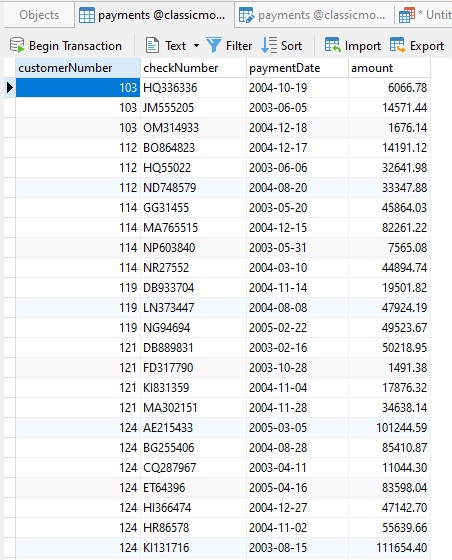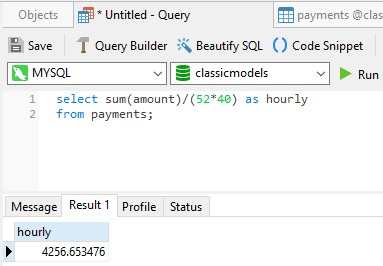# Navicat Blog

Floating Point Rounding Errors in MySQL Sep 3, 2021 by Robert Gravelle

Although MySQL DECIMAL and NUMERIC data types are both fixed-point values, they are still susceptible to rounding errors. The reason is that, no matter how many digits a type can accommodate (the maximum number of digits for DECIMAL is 65!) that number is still fixed. Moreover, DECIMAL columns can be assigned a precision or scale that could have the potential affect of truncation to the allowed number of digits.

I became aware of potential rounding errors in MySQL when a reader asked me why a couple of similar queries were returning slightly different DECIMAL values in calculations. This prompted me to go on a journey of discovery. In today's blog, I would like to share some of what I learned about floating point rounding in MySQL.

# A Tale of Two Queries

Here are the queries I used to show the discrepancy:

Note: some rows were removed from the Group By With Rollup query to reduce the height of the image.

The reader was calculating employee salaries, but I did not have an identical table to query, so I used the most similar table that I could find, and that was the payments table of the classicmodels sample database:In this context, perhaps calculating hourly payments does not make much sense, but the queries did highlight the rounding differences between the two SELECT statements (4256.653475 vs. 4256.653476).

So, why does SUM using a subquery and the GROUP BY WITH ROLLUP produce different results?

# Floating-point Approximate Values Versus Fixed-point Exact Values

The floating-point (approximate value) types are FLOAT, REAL, and DOUBLE, while the fixed-point (exact value) types are INTEGER, SMALLINT, DECIMAL, and NUMERIC. Floating-point means the decimal point can be placed anywhere relative to the significant digits of the number with the actual position being indicated separately. Meanwhile, a fixed-point value is an integer that is scaled by a specific factor.

Back in version 5.5, MySQL added support for precision math, which included a library for fixed-point arithmetic that replaced the underlying C library and allowed operations to be handled in the same manner across different platforms. Since this update, if no approximate values or strings are being used in a calculation, expressions are evaluated using DECIMAL exact value arithmetic with precision of 65 digits. For GROUP BY functions, STDDEV() and VARIANCE() return DOUBLE, an approximate floating-point type, while SUM() and AVG() return a DECIMAL for exact-value arguments and a DOUBLE for approximate value.

Another ramification of the new MySQL library for fixed-point arithmetic is that type conversion is now handled using floating-point values. Thus, the results of type conversion may vary and can be affected by factors such as computer architecture, the compiler version or even the optimization level. One way to avoid these problems is to use an explicit CAST() rather than the implicit conversion.

# Which Result is Correct?

Getting back to the initial queries, which value is more accurate and which one is the correct way to obtain the SUM? The truth is, neither is exactly accurate, but, by using a little algebra, the query can be simplified to yield an accurate result:The key to accurate rounding is to work with whole numbers in as many initial steps as possible.

Blog Archives
Share
• Partners